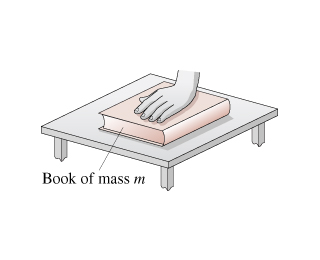# Problem: A hand presses down on the book in the figure.Is the normal force of the table on the book larger than, smaller than, or equal to mg?

###### FREE Expert Solution
83% (277 ratings)
###### Problem Details

A hand presses down on the book in the figure.

Is the normal force of the table on the book larger than, smaller than, or equal to mg?

Frequently Asked Questions

What scientific concept do you need to know in order to solve this problem?

Our tutors have indicated that to solve this problem you will need to apply the Vertical Equilibrium & The Normal Force concept. You can view video lessons to learn Vertical Equilibrium & The Normal Force. Or if you need more Vertical Equilibrium & The Normal Force practice, you can also practice Vertical Equilibrium & The Normal Force practice problems.